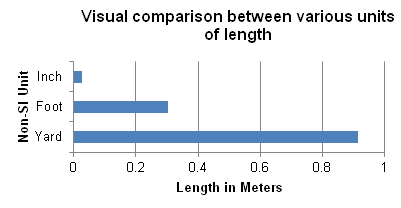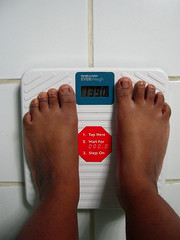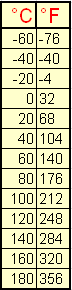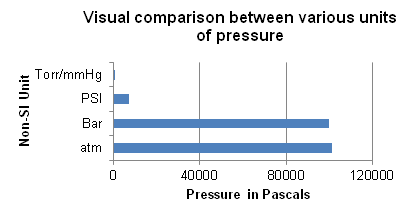# Non-SI Units

The metric system of measurement, the International system of units (SI Units), is widely used for quantitative measurements of matter in science and in most countries. However, different systems of measurement existed before the SI system was introduced. Any units used in other system of measurement (i.e. not included in the SI system of measurement), will be referred to as non-SI units. In most science courses, non-SI Units are not be used regularly.

## Introduction

Non-SI units existed long before the invention of SI units. However, these units have been replaced by the standard SI Units in most countries. The countries that have continued to use non-SI units, such as the United States, keep these units mainly for economic, business, and cultural reasons. The following table will introduce some of the commonly used non-SI units, as well as their SI counterparts:

 Non-SI Units and SI Unit List Physical Quantity Non-SI Unit(s) SI unit(s) Length Angstrom (Å), inch (in), feet (ft), yard (yd) meter (m) Mass atomic mass unit (amu), pound (lb), ounce (oz) kilogram (kg) Weight, Force pound (lb) Newton (N) Volume quart (qt), gallon (gal), pint (pt), fluid ounce (fl oz), cup Liter (L), cubic meter (m3) Temperature Fahrenheit (°F), Celsius (°C) Kelvin (K) Time Hour, year, unix time second (s) Energy Calorie (Cal), kilowatt-hour (kWh), electronvolt(eV) Joule (J) Pressure pounds per square inch (psi), atmospheres (atm), millimeters of mercury (mmHg), Barr, Torr Pascal (Pa)
*Only the most common units are listed; this list is not complete, and other units exist.

## Length: Meter (m)1 meter = 1010Å = 39.370 inches = 3.281 feet = 1.093 yards = 0.000621 miles

Inches, feet, and yards are used for quantities of length close to an everyday scale (the distance between tables, the distance to the door, the height of a person). Miles are used for larger distances and values of length (the height of buildings, distances to the next town, circumference of the Earth). You will probably not encounter these units in Chemistry. Note: feet and inches are sometimes denoted with ' and " respectively (e.g. 3 feet 8 inches 3'8").

Angstroms are usually used to measure things on an atomic scale (the radius of an atom, the distance between atoms, the size of a unit cell in a crystal lattice). You will encounter these units in the Chemistry classroom. Other units used to measure length and distance include Astronomical Units (AU), light years (ly), and parsecs (pc), used to measure great distances on the orders of magnitude of 109 and1016 meters. These units are used in certain physics classes, and will probably not be used in Chemistry.

The SI unit for length, the meter, can be and will be used instead of most of these, since the system of prefixes allows it to be very flexible.

## Mass: Kilogram (kg)1 kilogram = 6.022×1026 atomic mass units = 2.205 pounds = 35.274 ounces

Pounds are generally used in the United States (and other countries) as units of weight. However, they can and are also used as units of mass, sometimes referred to as mass-pounds to reduce confusion with the unit for force. Mass refers to the amount of matter in an object, while the weight refers to the downward force this object exerts in the presence of gravity. On Earth, the two values are the same. The pound and the ounce units will not likely be used in Chemistry.

Atomic mass units are the value that you see on the periodic table of elements under each element (it is also the weight of one mole of the substance in grams). It signifies how much one atom of the substance weighs.

The SI unit for mass, the kilogram, will be used in most calculations, while the gram (the kilogram without the prefix kilo), which is equal to 10-3 kilograms, will be used for most measurements and observations, since the quantities used in labs are generally not on the kilogram scale.

## Weight/Force: Newton (N)A person weighing 139 lb, or 618.303 N Image used under the Creative Commons License. [Source]

1 Newton = 0.224808943 pounds

Downward force in the presence of gravity is known as weight, so the two are related. Normally when people talk about pounds, they mean this downward force, or how much an object would weigh when put on a scale. However, pounds, sometimes called force-pounds to reduce confusion with the unit for mass, can also be used to describe any force (such as the force a beam puts on a wall, etc.).

In almost all cases, forces will be described in Newtons, the SI unit for force, equal to the force required to accelerate a mass of 1kg by 1m/s2.

Other units that can be used to measure force and weight include the poundal (pdl) and dyne (dyn).

The SI unit for mass, the kilogram, can also be used as a measure of force or weight. However, when used this way, it is commonly referred to as the kilogram-force (kgf) or the kilopond (kp). One kilopond is equal to the force exerted by a 1 kg mass in Earth's gravitational field (9.81 m/s2), or 9.81N.

## Volume: Liter (L)1 Liter = 1.057 quarts = 2.113 pints = 4.227 cups = 0.264 gallons = 33.814 fluid ounces

The quart, gallon, and fluid ounces are used commonly in countries that still use non-SI units to describe the volume of containers, such as milk jugs, soda bottles, and juice boxes. For conversion purposes, it is worth noting that one quart is almost equal to one liter, and one gallon is almost equal to four liters.

Pints are almost exclusively used when referring to alcohol (e.g. a pint of beer), and cups are used almost exclusively in cooking (e.g. a recipe that calls for a cup of milk and a cup of sugar).

The SI unit Liter is usually too big for most laboratory measurements. Therefore, milliliters (mL), the Liter with the SI prefix meaning 10-3, are commonly used instead. However, most formulas still use the Liter.

## Temperature: Kelvin (K)Conversion formulas for temperature:

$\text{F}=\dfrac{9}{5}\centerdot \text{C}+32$
$\text{C}=\dfrac{5}{9}\centerdot(\text{F}-32)=\text{K}-273.15$
$\text{K}=\text{C}+273.15$

The three scales of temperature are Fahrenheit, Celsius, and the SI Scale Kelvin. Since they are scales rather than quantities, formulas are used to convert from one to another. However, an increase by one °C is the same as an increase by one K. However, the same measurement will be in different places on different scales. For instance, at one atmosphere of pressure, water freezes at 0°C, 32°F, and 273K, and boils at 100°C, 212°F, and 373.15K.

Note: The SI unit Kelvin does not have a degree sign, therefore one would say "273 Kelvin" rather than "273 degrees Kelvin." Also, -40°C is equal to -40°F.

## Time: Seconds (s)A soviet era clock from a submarine. A second hand and an hour hand are present. Image used under the Creative Commons License. [Source]

1 second = 0.0167 minutes = 0.000278 hours = 1.15740741×10-5days = 3.16887646×10-8 years

Or, more usefully:

1 year = 365.242 days = 8 765.813 hours = 525 948.766 minutes = 31 556 926 seconds

Unlike the other units, these units are used all over the world along with other types of time keeping units, such as weeks, months, and seasons to keep track of where the Earth is, designate events, and do all sorts of other things that require time precision.

The SI Unit seconds will be used in most laboratories (along with minutes, 1 minute = 60 seconds), however, since most reactions, especially the reactions performed in Chemistry 2A - 2C do not require more than that. Hours might be necessary for some reactions, and half-life, in certain cases, will be given in days or years.

Other units and measurements of time are also used. For instance, Unix based operating systems use what is called Unix time, a string of numbers signifying the amount of seconds that have passed since January 1st, 1970.

## Energy: Joule (J)

1 Joule = 2.39×10−4 Calories = 2.778×10-7Kilowatt hours = 6.24150974×1018electronvolts

Calories are most commonly found on the labels of food products. A Calorie (with a capital C) is not the same as a calorie. One Calorie is actually 1000 calories, or 1 Cal = 1 kcal. A calorie is the amount of energy required to heat up a gram (mL) of water by 1°C, so a Calorie is the amount of energy required to hear 1 Liter or 1 kilogram of water by 1°C.

Kilowatt hours are most commonly found on energy bills and energy counters.

The SI Unit Joule will be used for most calculations requiring energy. Energy is a system's ability to do work.

## Pressure: Pascal (P)1 Pascal = 9.86923267×10−6 atmospheres = 1.0×10-5 Bar = 0.0075 Torr = 0.0075 millimeteres Mercury = 0.000145 pounds per square inch

The atmosphere will most commonly be used in Chemistry, as a lot of formulas require the pressure to be in atmospheres.

The pounds per square inch (psi) and Bar units are commonly used on things that require a pressure, such as bike and car tires. For instance, car tires are generally inflated to 34 psi, or 2.344 Bar. Bike tire pressures range widely depending on the the tire.